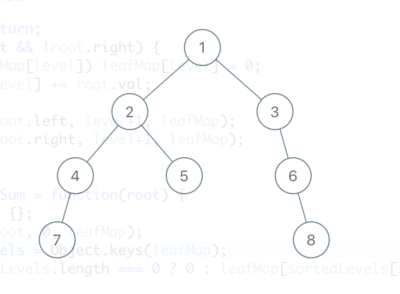## [Programming Problem] Deepest Leaves Sum

Given a binary tree, return the sum of values of its deepest leaves.

Example 1:Input: root = [1,2,3,4,5,null,6,7,null,null,null,null,8]
Output: 15

Algorithm

• Traverse through the tree using a level variable (which tells you which level you’re at).
• When you reach a leaf, maintain a key map of level->sum.
• In the end, pick the highest key from the map and output the value (as result).

Also TIL, that Property order is predictable in JavaScript objects since ES2015. Quoting from this article “All properties that are integer indices appear first in the overall object property order and are sorted numerically.”. This means we don’t need to sort the leaf map (by key) before returning the result.

```/** * Definition for a binary tree node. * function TreeNode(val, left, right) { * this.val = (val===undefined ? 0 : val) * this.left = (left===undefined ? null : left) * this.right = (right===undefined ? null : right) * } */ /** * @param {TreeNode} root * @return {number} */   var calcLeafMap = function(root, level, leafMap) { if (!root) return; if (!root.left && !root.right) { if (!leafMap[level]) leafMap[level] = 0; leafMap[level] += root.val; } calcLeafMap(root.left, level+1, leafMap); calcLeafMap(root.right, level+1, leafMap); };   var deepestLeavesSum = function(root) { let leafMap = {}; calcLeafMap(root, 0, leafMap); let sortedLevels = Object.keys(leafMap); return leafMap[sortedLevels[sortedLevels.length-1]]; };```

## [Programming Problem] Minimum Remove to Make Valid Parentheses

Given a string s of ‘(‘ , ‘)’ and lowercase English characters.

Your task is to remove the minimum number of parentheses ( ‘(‘ or ‘)’, in any positions ) so that the resulting parentheses string is valid and return any valid string.

Formally, a parentheses string is valid if and only if:

It is the empty string, contains only lowercase characters, or
It can be written as AB (A concatenated with B), where A and B are valid strings, or
It can be written as (A), where A is a valid string.

Example 1:
Input: s = “lee(t(c)o)de)”
Output: “lee(t(c)o)de”
Explanation: “lee(t(co)de)” , “lee(t(c)ode)” would also be accepted.

Example 2:
Input: s = “a)b(c)d”
Output: “ab(c)d”

Example 3:
Input: s = “))((”
Output: “”
Explanation: An empty string is also valid.

Example 4:
Input: s = “(a(b(c)d)”
Output: “a(b(c)d)”

Initial thought was do a BFS search where each outgoing node is the string without a bracket. At any level if we find a solution, we stop there (for e.g. in below example we would stop at Level 1 itself since we find “ab(c)d”).

``` "a)b(c)d"   "ab(c)d" ✔️ "a)bc)d" "a)b(cd"   "abc)d" "ab(cd" ... ...```

However, above solution will time out.

Instead we use the stack data structure to determine the brackets that cause invalidity of parentheses. These are simply the brackets remaining in the stack (at the end of validity test). With the bracket, we also store the index of the bracket (in the string) which makes it quicker to delete characters from those positions and rebuild valid string.

```/** * @param {string} s * @return {string} */   // Node representing bracket and its index (in the string) var Node = function(c, idx) { this.c = c; this.idx = idx; }   var minRemoveToMakeValid = function(s) { let sChars = Array.from(s);   // Use a stack to determine which brackets are causing 'invalidity' // We use Node data strcuture so that we know its index's (which makes it easy to remove) let stk = []; for ( let i = 0 ; i < sChars.length ; i++ ) { let curr = new Node(sChars[i], i); if ( curr.c === '(' || (curr.c === ')' && stk.length === 0) ) { stk.push(curr); } else if ( curr.c === ')' ) { let prev = stk.pop(); if ( prev.c === ')' ) { stk.push(prev); stk.push(curr); } } }   // index'es which we need to remove let toDelete = stk.map(function(n) { return n.idx; });   // build new string! let newS = ''; for ( let i = 0 ; i < sChars.length ; i++ ) { if (toDelete.includes(i)) continue; newS += sChars[i]; }   return newS; };```